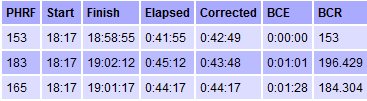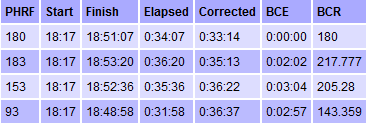# Understanding PHRF Results – TOT, BCE and BCR

Results for PHRF-handicapped races contain columns called BCR and BCE, which require some explanation. Before getting to that, it’s helpful to quickly review how PHRF handicapping works.
PHRF handicaps are based on seconds per nautical mile. For example, if boat A has a handicap of 150 and boat B has a handicap of 200, boat B is judged to be slower by 50 seconds per mile. This is a time-on-distance (TOD) approach. Since it is typically not practical to measure the length of a course and apply TOD handicapping, we use a time-on-time (TOT) approach instead. TOT works by converting a boat’s TOD handicap to a TOT factor, and then multiplying the elapsed time in seconds by the TOT factor to get the corrected time in seconds. To determine the TOT factor (TF), we plug the PHRF handicap into the following formula:

TOT Factor (TF) = 566.431 / (401.431 + PHRF)

For a handicap of 165, TF equals 1. For boats with lower handicaps (i.e. faster boats), TF is greater than 1, and for slower boats with higher handicaps, TF is less than 1. So for boats with a handicap of 165, the corrected time equals the elapsed time. For slower boats, corrected time is less than elapsed, and for faster boats, corrected time is greater than elapsed.

Here’s a fragment of some posted results:Note the relationship between corrected time and elapsed time, as explained above.

BCE and BCR
The above posted results also include columns labelled BCE and BCR, which often cause confusion. BCR stands for “back calculated rating”, which is the handicap you would have needed to tie the winner. For the winner, it matches the actual handicap, and for everyone else, it is higher than their actual handicap. In the example above, if the second boat had a handicap of 196.429 instead of 183, they would have tied for the win. We can check that by plugging 196.429 into the formula above to get a TOT factor of 0.947431. The elapsed time of 0:45:12 is 2712 seconds, and 2712 * 0.947431 is 2569 seconds, which equals 0:42:49, as required.

BCE stands for “back calculated elapsed”, which is how much faster you would have had to be on elapsed time to tie the winner. In the example above, BCE is 01:01 for the second boat. That would have given an elapsed time of 0:44:11, which is 2651 seconds. Their TOT factor is 0.969201, so corrected time would be 2651 * 0.969201, which is 2569 seconds (0:42:49) as required.

When BCE Looks Wrong
In the example above, BCE is greater for the third-place boat than it is for the second-place boat. Intuitively, it seems like the BCE should always increase for each successive position, but that’s not always the case.

As a simple example, suppose boat A has a TOT factor of 1.0 and boat B has a TOT factor of 0.5, and that boat A finishes with an elapsed time of 30:01 while B has an elapsed time of 60:00. Boat B’s corrected time would be 30:00, so she would finish one second ahead of A. Now suppose each boat’s elapsed time was reduced by 4 seconds, so A finished in 29:57 and B finished in 59:56. B’s corrected time would be 29:58, so their positions would switch. In other words, a 4 second decrease made a bigger difference to A than to B. So for A’s BCE to be equivalent to B’s, it would have to be smaller.
Here’s an example where this actually happened:The BCE for the fourth-place boat is less than the BCE for the third-place boat, because the fourth-place boat has a much faster handicap. If in doubt, do the math — it does work out!

Page maintained by H. Morrin.